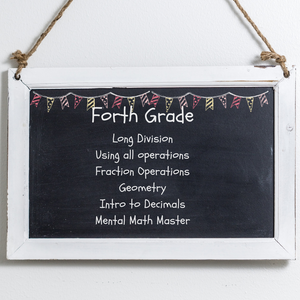# Club Genio (Age 9-10)

Regular price
\$59.99
Sale price
\$59.99
Regular price
Sold out
Unit price
per

Club Genio (Age 9-10)

We start our focus in this level to algebraic thinking.  We master multiplication up to 4 digit numbers and develop problem solving abilities using multiplication.

WHAT THEY'LL LEARN

Operations and Algebraic Thinking:

• Interpreting multiplication
• Meanings for multiplication
• Problem solving using multiplication
• Problem solving using missing factors
• Problem solving using division
• Problem solving with 3-digit numbers
• Multi-step word problems
• Using variable equations to solve word problems
• Prime vs. composite numbers
• Factors
• Problem solving with factors
• Problem solving with prime v. composite numbers
• Number patterns
• Shape patterns

Geometry

• Identify and draw points, lines, line segments, rays, angles, perpendicular and parallel lines
• Triangles: right, acute and obtuse
• Triangles: equilateral, isosceles, scalene
• Quadrilaterals: identify parallel and perpendicular lines
• Lines of symmetry

Numbers and Operations in Base 10:

• Place Value
• Base 10, Number Names & Expanded Form
• Greater than, less than, equal to
• Rounding
• Adding and Subtracting multi-digit whole numbers
• Adding and Subtracting problem solving
• Multiplication up to 4-digits
• Multiplication Problem Solving
• Division with and without remainders
• Division Problem Solving

Measurement and Data

• Measurement Units
• Measurement Conversion Tables
• Distance Word Problems
• Time Word Problems
• Volume Word Problems
• Money Word Problems
• Perimeter of rectangles
• Area of squares and rectangles
• Perimeter of rectangles
• Area Word Problems
• Area vs Perimeter Word Problems
• Line Plots
• Graphs
• Angles and Circles
• Measuring Angles with a Circle
• Measuring Angles with a Protractor
• Solving for Unknown Angles

Number and Operations-Fractions

• Equivalent Fractions
• Comparing Fractions
• Common Denominator
• Addition and Subtraction of Fractions
• Decomposing Fractions
• Adding and Subtracting Mixed Numbers
• Fraction Word Problems (Adding & Subtracting)
• Multiply Fractions & Whole Numbers
• Fraction Word Problems (Multiplication)
• Equivalent Fractions with Denominator as 10 and 100
• Decimals
• Comparing Decimals

Challenge your child with the educational miracle Club Genio Kids (Age: 9-10) and order yours online today!